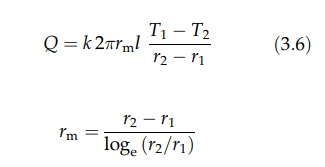# Heat Transfer in Pipes and Tubes

| Home | | Pharmaceutical Technology |

## Chapter: Pharmaceutical Engineering: Heat Transfer

Pipes and tubes are common barriers over which heat exchange takes place.

HEAT TRANSFER IN PIPES AND TUBES

Pipes and tubes are common barriers over which heat exchange takes place. Conduction is complicated in this case by the changing area over which heat is transferred. If equation (3.2) is to be retained, some value of A must be derived from the length of the pipe, l, and the internal and external radii, r1 and r2, respectively. When the pipe is thin walled and the ratio r2/r1 is less than approximately 1.5, the heat transfer area can be based on an arithmetic mean of the two radii. Equation (3.2) then becomesThis equation is inaccurate for thick-walled pipes. Heat transfer area must then be calculated from the logarithmic mean radius, rm. The equation for heat transfer is then